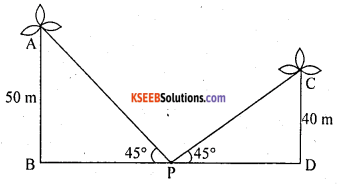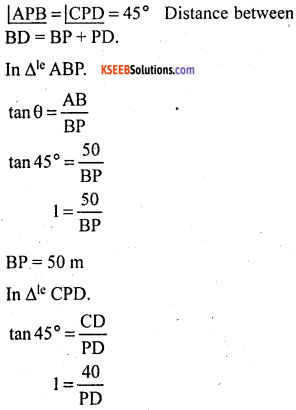# KSEEB Solutions for Class 10 Maths Chapter 12 Some Applications of Trigonometry Additional Questions

Students can Download Class 10 Maths Chapter 12 Some Applications of Trigonometry Additional Questions, Notes Pdf, KSEEB Solutions for Class 10 Maths helps you to revise the complete Karnataka State Board Syllabus and score more marks in your examinations.

## Karnataka State Syllabus Class 10 Maths Chapter 12 Some Applications of Trigonometry Additional Questions

I. Multiple Choice Questions:

Question 1.
The angle of elevation of the top of a tower from a point at a distance of 100 m from the base of the tower is found to be 45° then height of the tower is
a. 50 m
b. 100m
c. 50$$\sqrt{2}$$ m
d. 50$$\sqrt{2}$$ m
b. 100mQuestion 2.
Find the angle of elevation of the top of a tower, whose height is 100 m, at a point whose distance from the base of the tower is 100 m.
a. 30°
b. 60°
c. 45°
d. 90°
c. 45°

Question 3.
The angle of elevation of the top of tree from a point at a distance of 200 m from its base is 60° the height of the tree is
a. 50$$\sqrt{3}$$m
b. 100$$\sqrt{3}$$ m
c. 200$$\sqrt{3}$$m
d. $$\frac{200}{\sqrt{3}}$$ m
c. 200$$\sqrt{3}$$m

Question 4.
Find the length of the shadow of 10 m high tree of the angle of elevation of the sun is 30°
a. 10 m
b. $$\frac{10}{\sqrt{3}}$$ m
c. 10$$\sqrt{3}$$m
d. 20 m
d. 20 m

Question 5.
If the shadow of 10 m high tree is 10$$\sqrt{3}$$m then find the angle of elevation of the sun
a. 60°
b. 90°
c. 45°
d. 30°
c. 45°

Question 6.
The ratio of the length of a tree and its shadow is 1 : $$\frac{1}{\sqrt{3}}$$. The angle of the sun’s elevation is
a. 30°
b. 45°
c. 60°
d. 90°
c. 60°

Question 7.
The ratio of the length of a rod to its shadow is 1: $$\sqrt{3}$$. The angle of elevation of the sun is
a. 30°
b. 60°
c. 45°
d. 90°
a. 30°Question 8.
If the angle of elevation of the sun is 45°, then find the length of the shadow of a tower whose height is ‘h’m
a. $$\frac{h}{2}$$ m
b. h m
c. 2h m
d. h$$\sqrt{3}$$ m
b. h m

Question 9.
The angle of elevation of the sun is 45°. Then, the length of the shadow of a 12 m high tree is
a. 6$$\sqrt{3}$$ m
b. 12$$\sqrt{3}$$ m
c. $$\frac{12}{\sqrt{3}}$$ m
d. 12 m
d. 12 m

Question 10.
From a bridge, 25 m high, the angle of depression of a boat is 45°. Find the horizontal distance of the boat from the bridge.
a. 25 m
b. $$\frac{25}{2}$$
c. 50 m
d. 25$$\sqrt{3}$$ m
a. 25 m

Question 11.
If the angle of elevation of the sun is top 60°, then find the ratio of the height of a tree with its shadow.
a. $$\sqrt{3}$$ : 1
b. 1 : $$\sqrt{3}$$
c. 3 : 1
d. 1 : 3
a. $$\sqrt{3}$$ : 1

Question 1.
The angle of elevation of an aeroplane from a point on the ground is 45°. After a flight of 15 seconds horizontally, the angle of elevation changes to 30° if the aeroplane is flying at a constant height of 2500 m then find the speed of the plane.2500 + BD = 2500$$\sqrt{3}$$
BD = 2500$$\sqrt{3}$$ – 2500
BD = 2500($$\sqrt{3}$$ – 1)m
But BD = CE = 2500 ($$\sqrt{3}$$ – 1) mSpeed = 118.33.m / sec

Question 2.
From a point on the ground 40 m away from the foot of a tower, the angle of elevation of the top of a tower is 30° the angle of elevation of the top of water tank on top of the tower is 45°. Find
(i) height of the tower
(ii) depth of the tank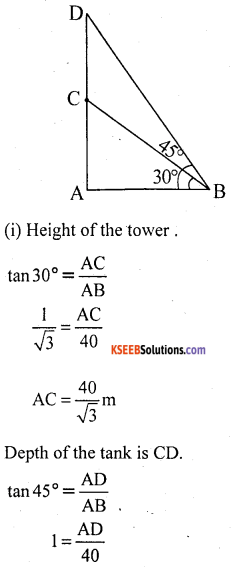∴ CD = AD – AC = 40 – $$\frac{40}{\sqrt{3}}$$
CD = 40$$\left(1-\frac{1}{\sqrt{3}}\right)$$ mQuestion 3.
A tall building casts a shadow of 300 m long when the sun’s altitude elevation is 30°. Find the height of the tower.= 100$$\sqrt{3}$$ m
Height of the tower is 100$$\sqrt{3}$$ m

Question 4.
A tree is broken over by the wind forms a right angled triangle with the ground. If the broken parts makes an angle of 60° with the ground and the top of the tree is now 20 m from its base, how tall was the tree.AB = 20$$\sqrt{3}$$ m.
Height of tree = AD = AB + BC
= 40 + 20$$\sqrt{3}$$
= 20(2 + $$\sqrt{3}$$)

Question 5.
The angle of elevation of the top of a flag post from a point on a horizontal ground is found to be 30°. On walking 6 m towards the post, the elevation increases by 15°.
Find the height of the flag post.In ∆ ABC θ = 30°.Y + 6 = x$$\sqrt{3}$$ → (1)
In ∆ ABD, θ = 45°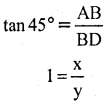x = y → (2)
Put equation (2) in equation (1)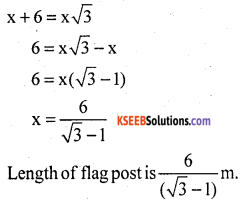Question 6.
From the top of a building 16 m high, the angular elevation of the top of a hill is 60° and the angular depression of the foot of the hill is 30°. Find the height of the hill.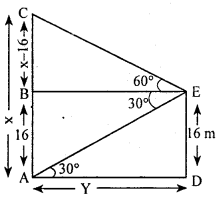In ∆ AED, θ = 30°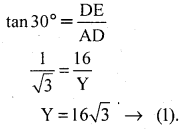In ∆ BCE, θ = 60°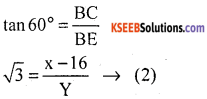From (1) and (2)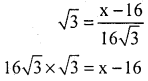16 × 3 = x – 16
x = 48 + 16 = 64
∴ The height of the hill is 64 m.

Question 7.
Two windmills of height 50 m and 40 m are on either side of the field. A person observes the top of the windmills from a point in between the towers. The angle of elevation was found to be 45° in both the cases. Find the distance between the windmills.